HomeRankingTask

Rank the total current from smallest to largest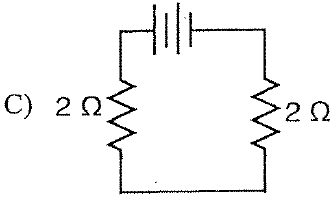Attaching Meters

School blocks YouTube? Use the file below.

Circuit Challenge

a) I1 = ?IT = I1 + I2

10.0 A =   I1 + 4.0 A

I1 = 6.0 A

b) VT = ?VT = V1 = V2

V2 = IR

= 4.0 A (30. ohms)

V2 = 120 V

So,

VT = 120 VAttaching MetersLearning-Connections Clipart

 Ammeter - Measures CurrentVoltmeter - Measures Voltage (Potential Difference) (= Energy Change/Coulomb)

Voltmeters
are connected 'in parallel'Ammeters
are connected 'in series'

 Find Ammeter, Voltmeter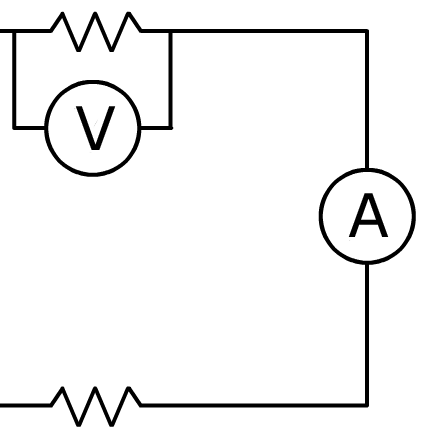Which circuit is correct?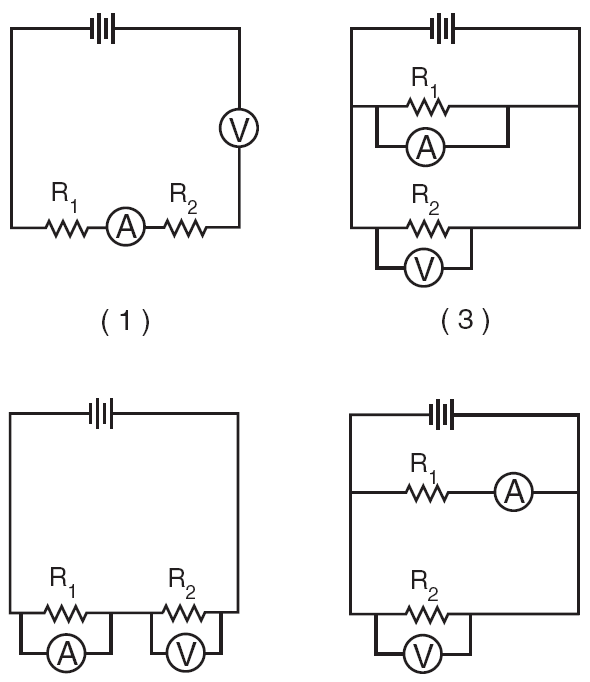June 2006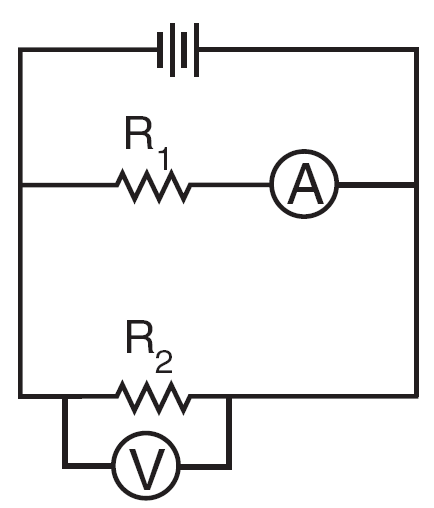Attaching Meters - Flash

[ HTML5 ]
St. Mary's H.S.

 Identify the switch in the circuit and circuit diagram shown below.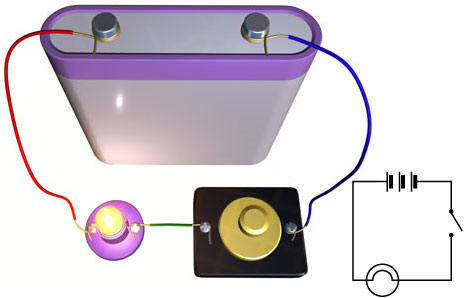Dorling Kindersley Books

Flash Animations for Physics
David M. HarrisonChoice 4Electric Power